# 交易机器人在市场发布前必须经过的检验

6 九月 2016, 18:40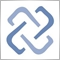1
3 716

### 怎样快速捕捉和修复交易机器人中的错误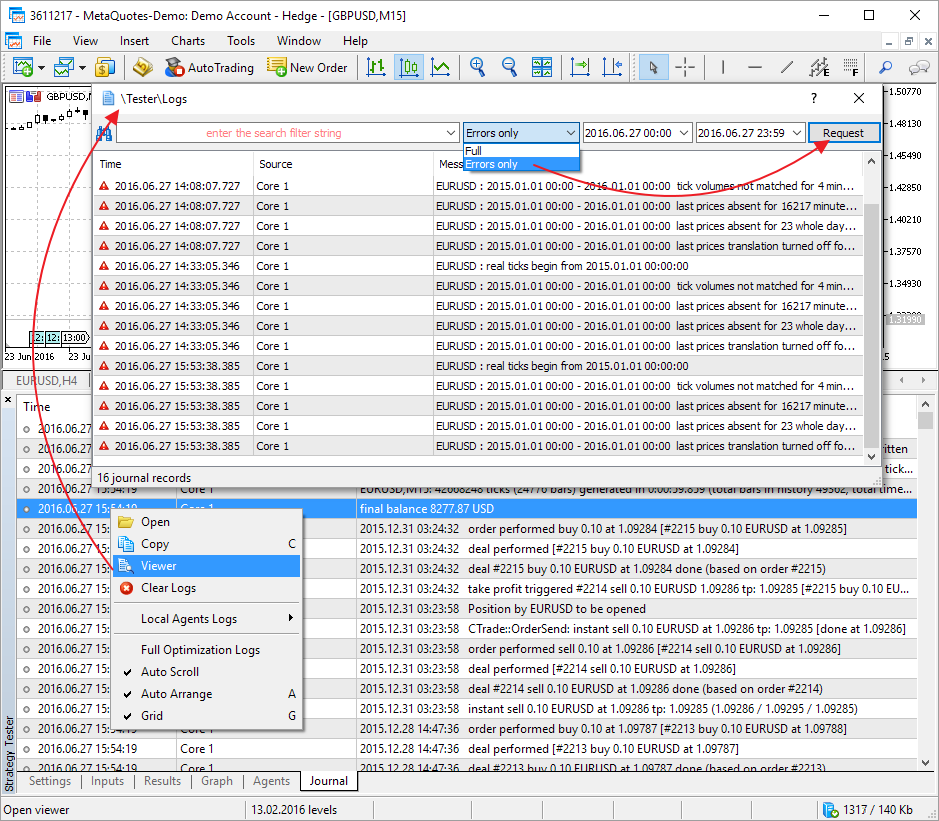### 资金不足以进行交易操作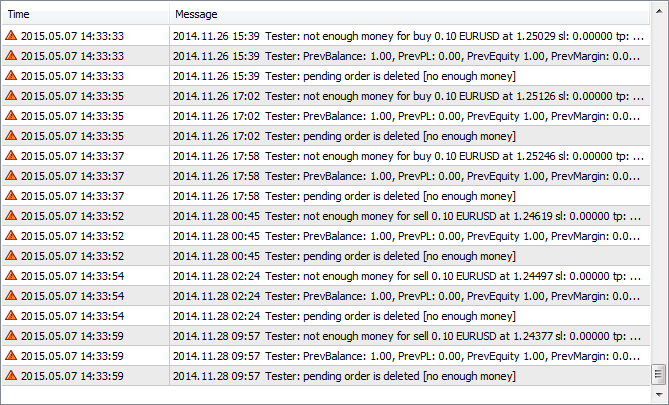MQL5

```bool CheckMoneyForTrade(string symb,double lots,ENUM_ORDER_TYPE type)
{
//--- 取得建仓价格
MqlTick mqltick;
SymbolInfoTick(symb,mqltick);
if(type==ORDER_TYPE_SELL)
price=mqltick.bid;
//--- 所需以及可用保证金的数值
double margin,free_margin=AccountInfoDouble(ACCOUNT_MARGIN_FREE);
//--- 调用检验函数
if(!OrderCalcMargin(type,symb,lots,price,margin))
{
//--- 出错了，发送报告并返回 false
Print("有错误出现在 ",__FUNCTION__," 编号=",GetLastError());
return(false);
}
//--- 如果资金不够进行操作
if(margin>free_margin)
{
//--- 报告错误并返回 false
Print("资金不足以进行 ",EnumToString(type)," ",lots," ",symb," 错误编号=",GetLastError());
return(false);
}
//--- 检验成功
return(true);
}

```

MQL4

```bool CheckMoneyForTrade(string symb, double lots,int type)
{
double free_margin=AccountFreeMarginCheck(symb,type, lots);
//-- 如果资金不够
if(free_margin<0)
{
Print("资金不足以进行", oper," ",lots, " ", symb, " 错误编号",GetLastError());
return(false);
}
//--- 检验成功
return(true);
}

```

### 交易操作中的无效交易量

 SYMBOL_VOLUME_MIN 交易的最小交易量 SYMBOL_VOLUME_MAX 交易的最大交易量 SYMBOL_VOLUME_STEP 执行交易时最小的交易量变化步长

```//+------------------------------------------------------------------+
//| 检查订单交易量的正确性                                               |
//+------------------------------------------------------------------+
bool CheckVolumeValue(double volume,string &description)
{
//--- 交易操作允许的最小交易量
double min_volume=SymbolInfoDouble(Symbol(),SYMBOL_VOLUME_MIN);
if(volume<min_volume)
{
description=StringFormat("交易量小于允许的最小交易量，SYMBOL_VOLUME_MIN=%.2f",min_volume);
return(false);
}

//--- 交易操作允许的最大交易量
double max_volume=SymbolInfoDouble(Symbol(),SYMBOL_VOLUME_MAX);
if(volume>max_volume)
{
description=StringFormat("交易量大于允许的最大交易量，SYMBOL_VOLUME_MAX=%.2f",max_volume);
return(false);
}

//--- 取得交易量变化的最小步长
double volume_step=SymbolInfoDouble(Symbol(),SYMBOL_VOLUME_STEP);

int ratio=(int)MathRound(volume/volume_step);
if(MathAbs(ratio*volume_step-volume)>0.0000001)
{
description=StringFormat("交易量不是最小交易步长的整数倍，SYMBOL_VOLUME_STEP=%.2f, 最接近的正确交易量是 %.2f",
volume_step,ratio*volume_step);
return(false);
}
description="正确的交易量数值";
return(true);
}

```

### 挂单的限制数量

```//+------------------------------------------------------------------+
//| 检查是否还允许设置订单                                               |
//+------------------------------------------------------------------+
bool IsNewOrderAllowed()
{
//--- 取得账户中允许设置的挂单数量
int max_allowed_orders=(int)AccountInfoInteger(ACCOUNT_LIMIT_ORDERS);

//--- 如果没有限制，返回 true; 您可以发送一个订单
if(max_allowed_orders==0) return(true);

//--- 如果我们达到这一行，说明有限制; 找出已经设置了多少挂单
int orders=OrdersTotal();

//--- 返回比较结果
return(orders<max_allowed_orders);
}

```

### 某特定交易品种的手数限制

```//+------------------------------------------------------------------+
//| 返回指定交易品种的仓位大小                                            |
//+------------------------------------------------------------------+
double PositionVolume(string symbol)
{
//--- 尝试根据交易品种选择仓位
bool selected=PositionSelect(symbol);
//--- 有仓位
if(selected)
//--- 返回仓位交易量
return(PositionGetDouble(POSITION_VOLUME));
else
{
//--- 选择仓位出错报告
Print(__FUNCTION__," 执行 PositionSelect() 失败，交易品种为 ",
symbol," 错误编号 ",GetLastError());
return(-1);
}
}

```

```double max_volume=SymbolInfoDouble(Symbol(),SYMBOL_VOLUME_LIMIT);
if(max_volume==0) volume=SymbolInfoDouble(Symbol(),SYMBOL_VOLUME_MAX);

```

```//+------------------------------------------------------------------+
//|  返回交易品种当前挂单的交易量                                         |
//+------------------------------------------------------------------+
double   PendingsVolume(string symbol)
{
double volume_on_symbol=0;
ulong ticket;
//---  获得全部交易品种当前下单数量
int all_orders=OrdersTotal();

//--- 恢复范围内的全部订单
for(int i=0;i<all_orders;i++)
{
//--- 获得列表中持仓的订单号
if(ticket=OrderGetTicket(i))
{
//--- 如果订单中指定我们的交易品种，增加该订单的交易量
if(symbol==OrderGetString(ORDER_SYMBOL))
volume_on_symbol+=OrderGetDouble(ORDER_VOLUME_INITIAL);
}
}
//--- 返回指定交易品种当前已下挂单的总交易量
return(volume_on_symbol);
}```

```//+------------------------------------------------------------------+
//| 返回交易品种中一个订单允许的最大交易量                                  |
//+------------------------------------------------------------------+
double NewOrderAllowedVolume(string symbol)
{
double allowed_volume=0;
//--- 取得订单最大交易量限制
double symbol_max_volume=SymbolInfoDouble(Symbol(),SYMBOL_VOLUME_MAX);
//--- 取得一个交易品种的交易量限制
double max_volume=SymbolInfoDouble(Symbol(),SYMBOL_VOLUME_LIMIT);

//--- 取得一个交易品种已建仓位的交易量
double opened_volume=PositionVolume(symbol);
if(opened_volume>=0)
{
//--- 如果我们已经用完了交易量
if(max_volume-opened_volume<=0)
return(0);

//--- 如果已建仓位的交易量没有超过 max_volume
double orders_volume_on_symbol=PendingsVolume(symbol);
allowed_volume=max_volume-opened_volume-orders_volume_on_symbol;
if(allowed_volume>symbol_max_volume) allowed_volume=symbol_max_volume;
}
return(allowed_volume);
}

```

### 在 SYMBOL_TRADE_STOPS_LEVEL 最小水平之内设置获利(TakeProfit)和止损(StopLoss)水平

• 买入是根据卖方报价(Ask)的 — 而止损和获利水平应该和买方报价(Bid)做比较。
• 卖出是根据买方报价(Bid)的 - 获利和止损水平应该和卖方报价(Ask)做比较。

TakeProfit >= Bid
StopLoss <= Bid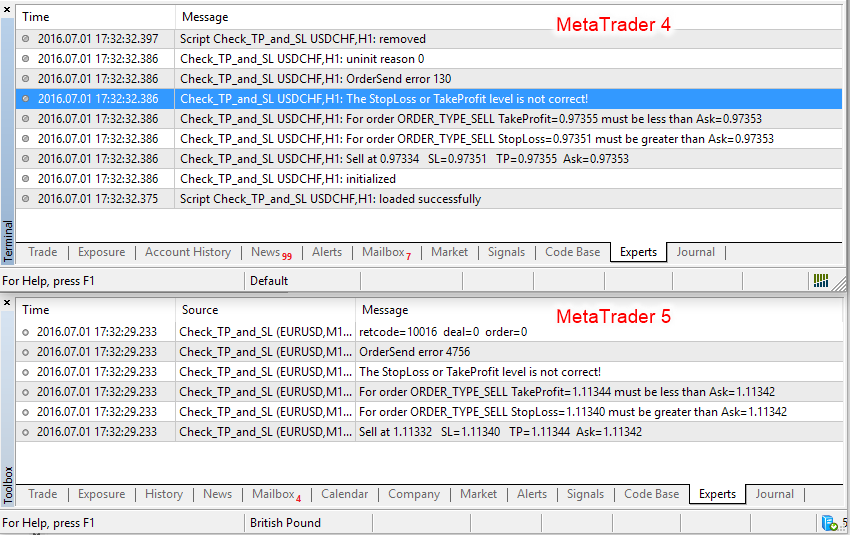• 买入是根据卖方报价的 — 获利和止损水平必须距离买方报价至少 SYMBOL_TRADE_STOPS_LEVEL 个点
• 卖出是根据买方报价的 — 获利和止损水平必须距离卖方报价至少 SYMBOL_TRADE_STOPS_LEVEL 个点

TakeProfit - Bid >= SYMBOL_TRADE_STOPS_LEVEL
Bid - StopLoss >= SYMBOL_TRADE_STOPS_LEVEL

```bool CheckStopLoss_Takeprofit(ENUM_ORDER_TYPE type,double SL,double TP)
{
//--- 取得 SYMBOL_TRADE_STOPS_LEVEL 水平
if(stops_level!=0)
{
" 距离当前价格大于 %d 个点",stops_level,stops_level);
}
//---
bool SL_check=false,TP_check=false;
//--- 只检查两种订单类型
switch(type)
{
//--- 买入操作
{
//--- 检验止损
SL_check=(Bid-SL>stops_level*_Point);
if(!SL_check)
PrintFormat("对于订单 %s 止损=%.5f 必须小于 %.5f"+
" (Bid=%.5f - SYMBOL_TRADE_STOPS_LEVEL=%d points)",
EnumToString(type),SL,Bid-stops_level*_Point,Bid,stops_level);
//--- 检验获利
TP_check=(TP-Bid>stops_level*_Point);
if(!TP_check)
PrintFormat("对于 %s 获利=%.5f 必须大于 %.5f"+
" (Bid=%.5f + SYMBOL_TRADE_STOPS_LEVEL=%d points)",
EnumToString(type),TP,Bid+stops_level*_Point,Bid,stops_level);
//--- 返回检验结果
return(SL_check&&TP_check);
}
//--- 卖出操作
case  ORDER_TYPE_SELL:
{
//--- 检验止损
if(!SL_check)
PrintFormat("对于订单 %s 止损=%.5f 必须大于 %.5f "+
//--- 检验获利
if(!TP_check)
PrintFormat("对于订单 %s 获利=%.5f 必须小于 %.5f "+
//--- 返回检验结果
return(TP_check&&SL_check);
}
break;
}
//--- 对于挂单有少许不同
return false;
}

```

```//+----------------------------------------+
//| 脚本程序起始函数                          |
//+----------------------------------------+
void OnStart()
{
//--- 随机取得操作类型
int oper=(int)(GetTickCount()%2); // 被2除永远余0或者1
switch(oper)
{
case  0:
{
//--- 取得建仓价格并且故意设置无效的获利/止损/
double SL=NormalizeDouble(Bid+2*_Point,_Digits);
double TP=NormalizeDouble(Bid-2*_Point,_Digits);
//--- 进行检查
PrintFormat("Buy at %.5f   SL=%.5f   TP=%.5f  Bid=%.5f",price,SL,TP,Bid);
Print("止损或者获利水平是不对的!");
//--- 还是尝试买入，是为了看执行结果
}
break;
//--- sell
case  1:
{
//--- 取得建仓价格并且故意设置无效的获利/止损/
double price=Bid;
//--- 进行检查
if(!CheckStopLoss_Takeprofit(ORDER_TYPE_SELL,SL,TP))
Print("止损或者获利水平是不对的!");
//--- try to sell anyway, in order to see the execution result
Sell(price,SL,TP);
}
break;
//---
}
}

```

```MQL5
Check_TP_and_SL (EURUSD,H1) Buy at 1.11433   SL=1.11425   TP=1.11421  Bid=1.11423
Check_TP_and_SL (EURUSD,H1) SYMBOL_TRADE_STOPS_LEVEL=30: 止损和获利距离平仓价格不能少于30个点
Check_TP_and_SL (EURUSD,H1) For order ORDER_TYPE_BUY 止损=1.11425 必须小于 1.11393 (Bid=1.11423 - SYMBOL_TRADE_STOPS_LEVEL=30 个点)
Check_TP_and_SL (EURUSD,H1) For order ORDER_TYPE_BUY 获利=1.11421 必须大于 1.11453 (Bid=1.11423 + SYMBOL_TRADE_STOPS_LEVEL=30 个点)
Check_TP_and_SL (EURUSD,H1) 止损或者获利水平是不对的!
Check_TP_and_SL (EURUSD,H1) OrderSend 错误 4756
Check_TP_and_SL (EURUSD,H1) retcode=10016  deal=0  order=0
MQL4
Check_TP_and_SL EURUSD,H1:  卖出于1.11430   SL=1.11445   TP=1.11449  卖方报价=1.11447
Check_TP_and_SL EURUSD,H1:  SYMBOL_TRADE_STOPS_LEVEL=1: 止损和获利水平与收盘价的距离不能少于1个点
Check_TP_and_SL EURUSD,H1:  对于订单 ORDER_TYPE_SELL 止损=1.11445 必须大于 1.11448  (买方报价=1.11447 + SYMBOL_TRADE_STOPS_LEVEL=1 个点)
Check_TP_and_SL EURUSD,H1:  对于订单 ORDER_TYPE_SELL 获利=1.11449 必须小于 1.11446  (卖方报价=1.11447 - SYMBOL_TRADE_STOPS_LEVEL=1 个点)
Check_TP_and_SL EURUSD,H1:  止损或者获利水平是不对的!
Check_TP_and_SL EURUSD,H1:  OrderSend 错误 130

```

Test_Wrong_StopLoss_LEVEL.mq5 例子EA交易的执行结果:

```Test_Wrong_StopLoss_LEVEL.mq5
Point=0.00001 Digits=5
1. 买入1.0 EURUSD 价格1.11442 SL=1.11404 Bid=1.11430 ( StopLoss-Bid=-26 个点 ))
CTrade::OrderSend: 立即买入 1.00 EURUSD 价格 1.11442 sl: 1.11404 [无效止损]
2. 买入1.0 EURUSD 价格1.11442 SL=1.11404 Bid=1.11431 ( StopLoss-Bid=-27 个点 ))
CTrade::OrderSend: 立即买入 1.00 EURUSD 价格 1.11442 sl: 1.11404 [无效止损]
3. 买入 1.0 EURUSD 价格 1.11442 SL=1.11402 Bid=1.11430 ( StopLoss-Bid=-28 个点 ))
CTrade::OrderSend: 立即买入 1.00 EURUSD 价格 1.11442 sl: 1.11402 [无效止损]
4. 买入 1.0 EURUSD 价格 1.11440 SL=1.11399 Bid=1.11428 ( StopLoss-Bid=-29 个点 ))
CTrade::OrderSend: 立即买入 1.00 EURUSD 价格 1.11440 sl: 1.11399 [无效止损]
5. Buy 1.0 EURUSD at 1.11439 SL=1.11398 Bid=1.11428 ( StopLoss-Bid=-30 points ))
Buy 1.0 EURUSD done at 1.11439 with StopLoss=41 points (spread=12 + SYMBOL_TRADE_STOPS_LEVEL=30)

```

Example of the Test_Wrong_TakeProfit_LEVEL.mq5 EA execution:

```Test_Wrong_TakeProfit_LEVEL.mq5
Point=0.00001 Digits=5
1. 买入1.0 EURUSD 价格 1.11461 TP=1.11478 Bid=1.11452 (TakeProfit-Bid=26 个点)
CTrade::OrderSend: 立即买入 1.00 EURUSD 价格 1.11461 tp: 1.11478 [无效止损]
2. 买入 1.0 EURUSD 价格 1.11461 TP=1.11479 Bid=1.11452 (TakeProfit-Bid=27个点)
CTrade::OrderSend: 立即买入 1.00 EURUSD 价格 1.11461 tp: 1.11479 [无效止损]
3. 买入1.0 EURUSD 价格 1.11461 TP=1.11480 Bid=1.11452 (TakeProfit-Bid=28个点)
CTrade::OrderSend: 立即买入 1.00 EURUSD 价格 1.11461 tp: 1.11480 [无效止损]
4. 买入1.0 EURUSD 价格1.11461 TP=1.11481 Bid=1.11452 (TakeProfit-Bid=29 个点)
CTrade::OrderSend: 立即买入 1.00 EURUSD 价格 1.11461 tp: 1.11481 [无效止损]
5. 买入1.0 EURUSD 价格1.11462 TP=1.11482 Bid=1.11452 (TakeProfit-Bid=30个点)

```

TakeProfit - Open >= SYMBOL_TRADE_STOPS_LEVEL
Open - StopLoss >= SYMBOL_TRADE_STOPS_LEVEL
Open - TakeProfit >= SYMBOL_TRADE_STOPS_LEVEL
StopLoss - Open >= SYMBOL_TRADE_STOPS_LEVEL

Test_StopLoss_Level_in_PendingOrders.mq5 EA 交易进行了一系列的止损买入和限价买入尝试，直到操作成功。在每次成功的尝试中，止损或者获利水平都在正确方向上偏移一个点。此EA交易执行的实例:

```Test_StopLoss_Level_in_PendingOrders.mq5
1. 止损买入 1.0 EURUSD 价格 1.11019 SL=1.10993 (Open-StopLoss=26 个点)
CTrade::OrderSend: 止损买入 1.00 EURUSD 价格 1.11019 sl: 1.10993 [无效止损]
2. 止损买入1.0 EURUSD 价格 1.11019 SL=1.10992 (Open-StopLoss=27 个点)
CTrade::OrderSend: 止损买入 1.00 EURUSD 价格 1.11019 sl: 1.10992 [无效止损]
3. 止损买入 1.0 EURUSD 价格 1.11020 SL=1.10992 (Open-StopLoss=28 个点)
CTrade::OrderSend: 止损买入 1.00 EURUSD 价格 1.11020 sl: 1.10992 [无效止损]
4. 止损买入 1.0 EURUSD 价格 1.11021 SL=1.10992 (Open-StopLoss=29 个点)
CTrade::OrderSend: 止损买入 1.00 EURUSD 价格 1.11021 sl: 1.10992 [无效止损]
5. 止损买入 1.0 EURUSD 价格 1.11021 SL=1.10991 (Open-StopLoss=30 个点)

---------
1. 限价买入 1.0 EURUSD 价格 1.10621 TP=1.10647 (TakeProfit-Open=26 个点)
CTrade::OrderSend: 限价买入1.00 EURUSD 价格 1.10621 tp: 1.10647 [无效止损]
2. 限价买入1.0 EURUSD 价格 1.10621 TP=1.10648 (TakeProfit-Open=27 个点)
CTrade::OrderSend: 限价买入1.00 EURUSD 价格1.10621 tp: 1.10648 [无效止损]
3. 限价买入1.0 EURUSD 价格1.10621 TP=1.10649 (TakeProfit-Open=28 个点)
CTrade::OrderSend: 限价买入1.00 EURUSD 价格1.10621 tp: 1.10649 [无效止损]
4. 限价买入1.0 EURUSD 价格1.10619 TP=1.10648 (TakeProfit-Open=29个点)
CTrade::OrderSend: 限价买入1.00 EURUSD 价格 1.10619 tp: 1.10648 [无效止损]
5. 限价买入 1.0 EURUSD 价格1.10619 TP=1.10649 (TakeProfit-Open=30个点)

```

### 尝试在 SYMBOL_TRADE_FREEZE_LEVEL 水平范围之内修改订单或者仓位

```//--- 检查订单类型
switch(type)
{
//--- 限价买入挂单
{
//--- 检查当前价格与激活价格的距离
if(!check)
PrintFormat("订单 %s #%d 不能被修改: Ask-Open=%d 个点 < SYMBOL_TRADE_FREEZE_LEVEL=%d 个点",
return(check);
}
//--- 限价买入挂单
case  ORDER_TYPE_SELL_LIMIT:
{
//--- 检查当前价格与激活价格的距离
check=((price-Bid)>freeze_level*_Point);
if(!check)
PrintFormat("订单 %s #%d 不能被修改: Open-Bid=%d 个点 < SYMBOL_TRADE_FREEZE_LEVEL=%d 个点",
EnumToString(type),ticket,(int)((price-Bid)/_Point),freeze_level);
return(check);
}
break;
{
//--- 检查当前价格与激活价格的距离
if(!check)
PrintFormat("订单 %s #%d 不能被修改: Ask-Open=%d 个点 < SYMBOL_TRADE_FREEZE_LEVEL=%d 个点",
return(check);
}
//--- 止损卖出挂单
case  ORDER_TYPE_SELL_STOP:
{
//--- 检查当前价格与激活价格的距离
check=((Bid-price)>freeze_level*_Point);
if(!check)
PrintFormat("订单 %s #%d 不能被修改: Bid-Open=%d points < SYMBOL_TRADE_FREEZE_LEVEL=%d 个点",
EnumToString(type),ticket,(int)((Bid-price)/_Point),freeze_level);
return(check);
}
break;
}

```

### 当操作缺少历史报价的交易品种时发生错误

1. 程序检查所需的历史是否足够长，如果柱数少于所需，程序会请求缺少的数据，并在下一个订单时刻来临之前结束操作。这种方法是最正确的，它可以帮助避免很多错误，例如超出数组返回或者除零错误；
2. 程序不做任何检查，立即开始工作，就如同所有所需的交易品种和时段信息在请求时已经可用了，这种方法是有问题的，可能会带来许多无法预料的错误。

### 超出数组范围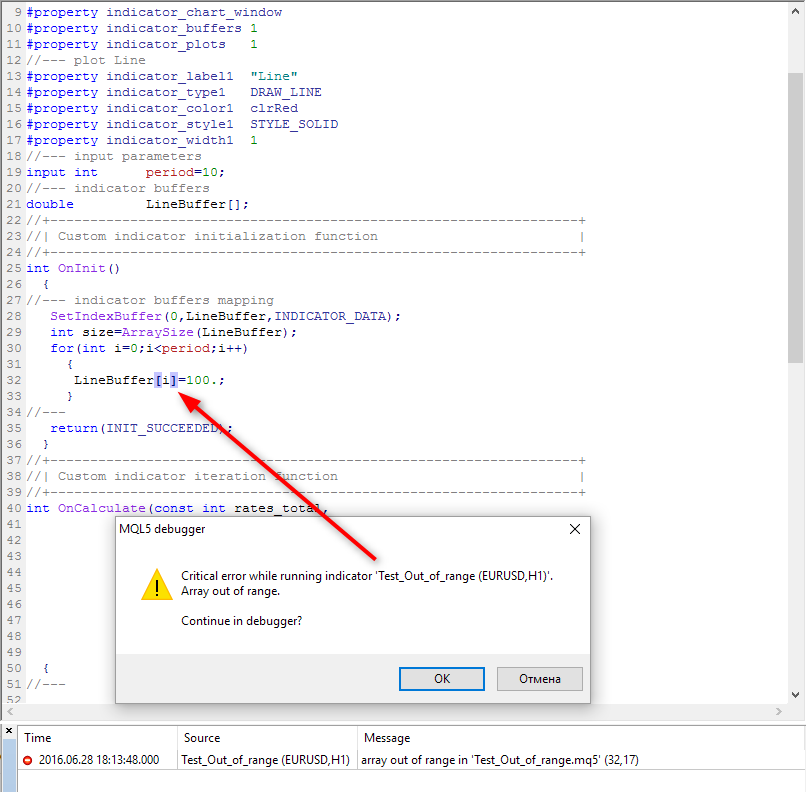### 除以零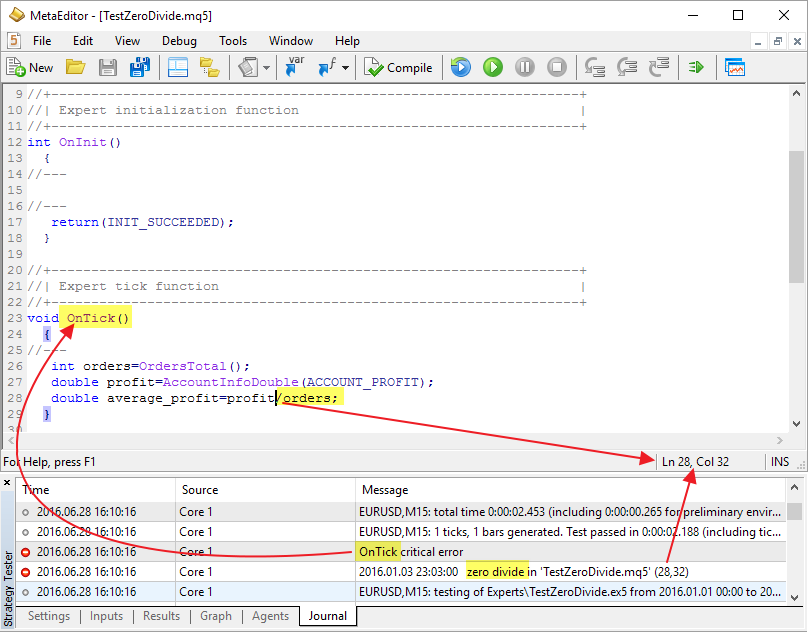### 发送请求来修改水平而并没有真正改变它们

```//--- 用于进行交易操作的类
//--- 用于操作订单的类
COrderInfo orderinfo;
//--- 用于操作仓位的类
CPositionInfo positioninfo;
//+------------------------------------------------------------------+
//| 在修改订单之前检查水平新的数值                                         |
//+------------------------------------------------------------------+
bool OrderModifyCheck(ulong ticket,double price,double sl,double tp)
{
//--- 根据编号选择订单
if(orderinfo.Select(ticket))
{
//--- 用于设置挂单的交易品种的点位大小和名称
string symbol=orderinfo.Symbol();
double point=SymbolInfoDouble(symbol,SYMBOL_POINT);
int digits=(int)SymbolInfoInteger(symbol,SYMBOL_DIGITS);
//--- 检查建仓价格有没有改变
bool PriceOpenChanged=(MathAbs(orderinfo.PriceOpen()-price)>point);
//--- 检查止损水平有没有改变
bool StopLossChanged=(MathAbs(orderinfo.StopLoss()-sl)>point);
//--- 检查获利水平有没有改变
bool TakeProfitChanged=(MathAbs(orderinfo.TakeProfit()-tp)>point);
//--- 如果水平有任何变化
if(PriceOpenChanged || StopLossChanged || TakeProfitChanged)
return(true);  // 订单可以修改
//--- 开盘价，止损和获利水平没有变化
else
//--- 通知错误
PrintFormat("订单 #%d 水平已经是 Open=%.5f SL=%.5f TP=%.5f",
ticket,orderinfo.PriceOpen(),orderinfo.StopLoss(),orderinfo.TakeProfit());
}
//--- 结束，订单没有改变
return(false);       // 不需要做修改
}
//+------------------------------------------------------------------+
//| 在修改订单之前检查水平新的数值                                        |
//+------------------------------------------------------------------+
bool PositionModifyCheck(ulong ticket,double sl,double tp)
{
//--- 根据编号选择订单
if(positioninfo.SelectByTicket(ticket))
{
//--- 用于设置挂单的交易品种的点位大小和名称
string symbol=positioninfo.Symbol();
double point=SymbolInfoDouble(symbol,SYMBOL_POINT);
//--- 检查止损水平有没有改变
bool StopLossChanged=(MathAbs(positioninfo.StopLoss()-sl)>point);
//--- 检查获利水平有没有改变
bool TakeProfitChanged=(MathAbs(OrderTakeProfit()-tp)>point);
//--- 如果水平有任何变化
if(StopLossChanged || TakeProfitChanged)
return(true);  // 仓位可以修改
//--- 止损和获利水平没有变化
else
//--- 通知错误
PrintFormat("订单 #%d 水平已经是 Open=%.5f SL=%.5f TP=%.5f",
ticket,orderinfo.PriceOpen(),orderinfo.StopLoss(),orderinfo.TakeProfit());
}
//--- 结束，订单没有改变
return(false);       // 不需要做修改
}
//+------------------------------------------------------------------+
//| 脚本程序起始函数                                                    |
//+------------------------------------------------------------------+
void OnStart()
{
//--- 订单和仓位的价格水平
double priceopen,stoploss,takeprofit;
//--- 当前订单和仓位的编号
ulong orderticket,positionticket;
/*
... 取得订单编号和新的止损/获利/建仓价格水平
*/
//--- 在修改挂单之前检查水平
if(OrderModifyCheck(orderticket,priceopen,stoploss,takeprofit))
{
//--- 检验成功
orderinfo.TypeTime(),orderinfo.TimeExpiration());
}
/*
... 取得仓位的编号和新的止损/获利水平
*/
//--- 在修改仓位之前做检查
if(PositionModifyCheck(positionticket,stoploss,takeprofit))
{
//--- 检验成功
}
//---
}

```

```#property strict
//+------------------------------------------------------------------+
//| 在修改订单之前检查水平新的数值                                         |
//+------------------------------------------------------------------+
bool OrderModifyCheck(int ticket,double price,double sl,double tp)
{
//--- 根据编号选择订单
if(OrderSelect(ticket,SELECT_BY_TICKET))
{
//--- 用于设置挂单的交易品种的点位大小和名称
string symbol=OrderSymbol();
double point=SymbolInfoDouble(symbol,SYMBOL_POINT);
//--- 检查建仓价格有没有改变
bool PriceOpenChanged=true;
int type=OrderType();
{
PriceOpenChanged=(MathAbs(OrderOpenPrice()-price)>point);
}
//--- 检查止损水平有没有改变
bool StopLossChanged=(MathAbs(OrderStopLoss()-sl)>point);
//--- 检查获利水平有没有改变
bool TakeProfitChanged=(MathAbs(OrderTakeProfit()-tp)>point);
//--- 如果水平有任何变化
if(PriceOpenChanged || StopLossChanged || TakeProfitChanged)
return(true);  // 订单可以修改
//--- 开盘价，止损和获利水平没有变化
else
//--- 通知错误
PrintFormat("订单 #%d 水平已经是 Open=%.5f SL=%.5f TP=%.5f",
ticket,OrderOpenPrice(),OrderStopLoss(),OrderTakeProfit());
}
//--- 结束，订单没有改变
return(false);       // 不需要做修改
}
//+----------------------------------------+
//| 脚本程序起始函数                          |
//+----------------------------------------+
void OnStart()
{
//--- 订单和仓位的价格水平
double priceopen,stoploss,takeprofit;
//--- 当前订单的编号
int orderticket;
/*
... 取得订单编号和新的止损/获利/建仓价格水平
*/
//--- 在修改订单之前做检查
if(OrderModifyCheck(orderticket,priceopen,stoploss,takeprofit))
{
//--- 检验成功
OrderModify(orderticket,priceopen,stoploss,takeprofit,OrderExpiration());
}
}

```

### 向函数传入无效参数(运行时错误)

ERR_INTERNAL_ERROR 4001 未料到的内部错误
ERR_WRONG_INTERNAL_PARAMETER 4002 使用错误参数调用客户终端内部函数
ERR_INVALID_PARAMETER 4003 使用错误参数调用系统函数
ERR_BUFFERS_WRONG_INDEX 4602 错误的指标缓冲区索引
ERR_INDICATOR_WRONG_PARAMETERS 4808 当创建指标时使用的参数数量错误
ERR_INDICATOR_PARAMETERS_MISSING 4809 创建指标时没有参数
ERR_INDICATOR_CUSTOM_NAME 4810 数组中的第一个参数必须是自定义指标的名称
ERR_INDICATOR_PARAMETER_TYPE 4811 当创建指标时数组中的参数类型不正确
ERR_NO_STRING_DATE 5030 字符串中没有日期
ERR_WRONG_STRING_DATE 5031 字符串中日期错误
ERR_TOO_MANY_FORMATTERS 5038 格式符号多于参数数量
ERR_TOO_MANY_PARAMETERS 5039 参数数量多于格式符号数量

### 访问冲突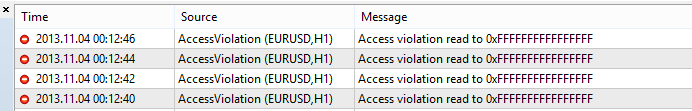### 消耗CPU资源和内存

```//+-----------------------------------------------+
//| 变化速率(Rate of Change, ROC)                   |
//+-----------------------------------------------+
int OnCalculate(const int rates_total,const int prev_calculated,const int begin,const double &price[])
{
//--- 检查数据数量
if(rates_total<ExtRocPeriod)
return(0);
//--- 计算开始时间
ulong start=GetMicrosecondCount();
//--- 预先计算
int pos=prev_calculated-1; // 设置计算位置
if(pos<ExtRocPeriod)
pos=ExtRocPeriod;
//--- 计算的主循环
for(int i=pos;i<rates_total && !IsStopped();i++)
{
if(price[i]==0.0)
ExtRocBuffer[i]=0.0;
else
ExtRocBuffer[i]=(price[i]-price[i-ExtRocPeriod])/price[i]*100;
}
//--- 计算结束时间
ulong finish=GetMicrosecondCount();
PrintFormat("Function %s in %s took %.1f ms",__FUNCTION__,__FILE__,(finish-start)/1000.);
//--- OnCalculate 完成返回新的 prev_calculated.
return(rates_total);
}

```

EA交易工作于它们自己的线程中，但是以上知识也同样应用于它们。写出优化的代码对于任何类型的程序都是重要的，不论是EA交易，指标，开发库或是脚本程序。

### 不能有过多的检验

2555_en.zip (26.47 KB)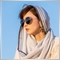| 30 6月 2017 在 09:09

very good!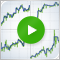交易者的 LifeHack: 四次回测比一次好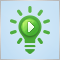交易者的 LifeHack: 测试中的余额，回撤，负载和订单指标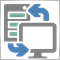在MQL中操作套接字，或者如何成为信号提供者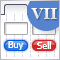图形界面 VII: 表格控件 (第一章)